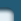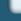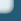Semigroups

Web design by Alexei Kuntsevich## Selected Publications

### Keyword(s): semigroups


F. Kappel. Semigroups and delay equations. In H. Brezis, M. G. Crandall, and F. Kappel, editors, Semigroups, Theory and Applications, Vol II, volume 152, pages 136–176, Harlow, 1986. Longman.


H. T. Banks and F. Kappel. Transformation semigroups and L1-approximation for size structured population models. Semigroup Forum, 38:141–155, 1989.


H. T. Banks, F. Kappel, and C. Wang. A semigroup formulation of a nonlinear size-structured distributed rate population model. In W. Desch, F. Kappel, and K. Kunisch, editors, Control and Estimation of Distributed Parameter Systems: Nonlinear Phenomena, volume 118 of ISNM (International Series of Numerical Mathematics), pages 1–19, Basel, 1994. Birkhäuser.


K. Ito and F. Kappel. Approximation of semilinear equations. Nonlinear Analysis: Theory, Methods and Applications, 24:51–80, 1995.

In this paper we prove well-posedness and approximation results for semilinear evolution equations in Banach spaces. These results are applicable to nonlinear delay equations with state and time dependent delays. As an example we consider a model in epidemics with threshold effects in the mechanism of infection.


F. Kappel. The Trotter-Kato theorem and approximation of abstract Cauchy problems. In Bambang Soedijono Subanar and Sri Wahyuni, editors, Proceedings of the Mathematical Analysis and Statistics Conference, Yogyakarta 1995, Part A: Theory and Methods, pages 50–58, 1996. ISBN 979-95118-0-1.


K. Ito and F. Kappel. The Trotter-Kato theorem and approximation of PDEs. Math. Computation, 67:21–44, 1998.

We present formulations of the Trotter-Kato theorem for approximation of linear C0-semigroups which provide very useful framework when convergence of numerical approximations to solutions of PDEs are studied. Applicability of our results is demonstrated using a first order hyperbolic equation, a wave equation and Stokes' equation as illustrative examples.


Abd El Rahman Aly Hussein and F. Kappel. The Trotter-Kato theorem and collocation methods for parabolic equations. In Sri Wahyuni, Retantyo Wardoyo, CH. Rini Indrati, and Supama, editors, Proc. of the SEAMS–GMU Internatl. Conf. Math. and its Appl., Yogyakarta, July 26–29, 1999, pages 22–36, Yogyakarta, Indonesia, 2000. Department of Mathematics, Gadjah Mada University. ISBN 979–95118–2--8.

Convergence of a C1 collocation scheme for a parabolic PDE in one space dimension is established for any initial data in the state space L2(0,1). The proof of converngence is based on the Trotter-Kato approximation scheme for C0-semigroups.


Abd El Rahman Aly Hussein, K. Ito, and F. Kappel. Some aspects of the Trotter-Kato approximation theorem for semigroups. In I. I. Eremin, I. Lasicka, and V. I. Maksimov, editors, Distributed Systems: Optimization and Economic-Environmental Applications (DSO'2000), Proceedings of an Internatl. Conf., May 30 – June 2, 2000, Ekaterinburg, Russia, pages 36–37, 2000. ISBN 5–7691–1047–3.

The classical proof of the Trotter-Kato theorems also provides rate estimates for smooth initial data. We discuss the possibilities to reduce the socalled smoothness gap for special types of semigroups as for instance analytic semigroups. Furthermore, we show that the assumption of stability of the approximating semigroups can be relaxed if at the same time the consistency requirement is replaced by a stronger assumption. Finally, we give a proof for a `folk' theorem for nonhomogeneous problems which repeatedly was proved under stronger assumptions than necessary.


K. Ito and F. Kappel. Locally quasi-dissipative evolution equations and applications to delay equations. submitted.

In this paper we discuss applications of the generation theory of nonlinear semigroups for evolution equations with locally quasi-dissipative operators in the sense of Kobayashi and Oharu to delay differential equations.